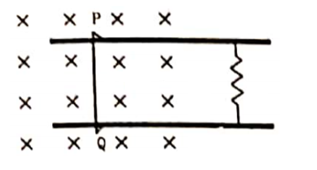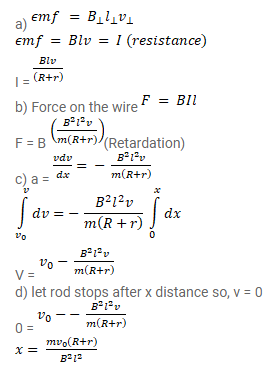# Consider the situation shown in figure.

Question:

Consider the situation shown in figure. The wire $\mathrm{PQ}$ has mass $\mathrm{m}$, resistance $\mathrm{r}$ and can slide on the smooth, horizontal parallel rails separated by a distance $\mathrm{I}$. The resistance of the rails is negligible. A uniform magnetic field $B$ exists in the rectangular region and a resistance $\mathrm{R}$ connects the rails outside the field region. At $\mathrm{t}=0$, the wire $\mathrm{PQ}$ is pushed towards right with the speed $^{v_{0}}$. Find

(a) the current in the loop at an instant when the speed of the wire $\mathrm{PQ}$ is $\mathrm{V}$.

(b) the acceleration of the wire at this instant,

(c) the velocity $v$ as a function of $x$ and

(d) the maximum distance the wire will move.Solution: